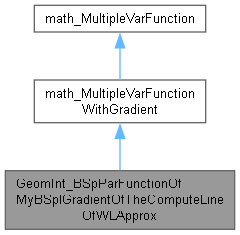`#include <GeomInt_BSpParFunctionOfMyBSplGradientOfTheComputeLineOfWLApprox.hxx>`[legend]

## Public Member Functions

GeomInt_BSpParFunctionOfMyBSplGradientOfTheComputeLineOfWLApprox (const GeomInt_TheMultiLineOfWLApprox &SSP, const Standard_Integer FirstPoint, const Standard_Integer LastPoint, const Handle< AppParCurves_HArray1OfConstraintCouple > &TheConstraints, const math_Vector &Parameters, const TColStd_Array1OfReal &Knots, const TColStd_Array1OfInteger &Mults, const Standard_Integer NbPol)
initializes the fields of the function. The approximating curve has <NbPol> control points. More...

Standard_Integer NbVariables () const
returns the number of variables of the function. It corresponds to the number of MultiPoints. More...

Standard_Boolean Value (const math_Vector &X, Standard_Real &F)
this method computes the new approximation of the MultiLine SSP and calculates F = sum (||Pui - Bi*Pi||2) for each point of the MultiLine. More...

Standard_Boolean Gradient (const math_Vector &X, math_Vector &G)
returns the gradient G of the sum above for the parameters Xi. More...

Standard_Boolean Values (const math_Vector &X, Standard_Real &F, math_Vector &G)
returns the value F=sum(||Pui - Bi*Pi||)2. returns the value G = grad(F) for the parameters Xi. More...

const math_VectorNewParameters () const
returns the new parameters of the MultiLine. More...

AppParCurves_MultiBSpCurve CurveValue ()
returns the MultiBSpCurve approximating the set after computing the value F or Grad(F). More...

Standard_Real Error (const Standard_Integer IPoint, const Standard_Integer CurveIndex)
returns the distance between the MultiPoint of range IPoint and the curve CurveIndex. More...

Standard_Real MaxError3d () const
returns the maximum distance between the points and the MultiBSpCurve. More...

Standard_Real MaxError2d () const
returns the maximum distance between the points and the MultiBSpCurve. More...

const math_MatrixFunctionMatrix () const
returns the function matrix used to approximate the multiline. More...

const math_MatrixDerivativeFunctionMatrix () const
returns the derivative function matrix used to approximate the multiline. More...

const math_IntegerVectorIndex () const
Returns the indexes of the first non null values of A and DA. The values are non null from Index(ieme point) +1 to Index(ieme point) + degree +1. More...

AppParCurves_Constraint FirstConstraint (const Handle< AppParCurves_HArray1OfConstraintCouple > &TheConstraints, const Standard_Integer FirstPoint) const

AppParCurves_Constraint LastConstraint (const Handle< AppParCurves_HArray1OfConstraintCouple > &TheConstraints, const Standard_Integer LastPoint) const

void SetFirstLambda (const Standard_Real l1)

void SetLastLambda (const Standard_Real l2)Public Member Functions inherited from math_MultipleVarFunction
virtual Standard_Integer GetStateNumber ()
return the state of the function corresponding to the latestt call of any methods associated to the function. This function is called by each of the algorithms described later which define the function Integer Algorithm::StateNumber(). The algorithm has the responsibility to call this function when it has found a solution (i.e. a root or a minimum) and has to maintain the association between the solution found and this StateNumber. Byu default, this method returns 0 (which means for the algorithm: no state has been saved). It is the responsibility of the programmer to decide if he needs to save the current state of the function and to return an Integer that allows retrieval of the state. More...

virtual ~math_MultipleVarFunction ()

## Protected Member Functions

void Perform (const math_Vector &X)
this method is used each time Value or Gradient is needed. More...

## Constructor & Destructor Documentation

 GeomInt_BSpParFunctionOfMyBSplGradientOfTheComputeLineOfWLApprox::GeomInt_BSpParFunctionOfMyBSplGradientOfTheComputeLineOfWLApprox ( const GeomInt_TheMultiLineOfWLApprox & SSP, const Standard_Integer FirstPoint, const Standard_Integer LastPoint, const Handle< AppParCurves_HArray1OfConstraintCouple > & TheConstraints, const math_Vector & Parameters, const TColStd_Array1OfReal & Knots, const TColStd_Array1OfInteger & Mults, const Standard_Integer NbPol )

initializes the fields of the function. The approximating curve has <NbPol> control points.

## ◆ CurveValue()

returns the MultiBSpCurve approximating the set after computing the value F or Grad(F).

## ◆ DerivativeFunctionMatrix()

 const math_Matrix& GeomInt_BSpParFunctionOfMyBSplGradientOfTheComputeLineOfWLApprox::DerivativeFunctionMatrix ( ) const

returns the derivative function matrix used to approximate the multiline.

## ◆ Error()

 Standard_Real GeomInt_BSpParFunctionOfMyBSplGradientOfTheComputeLineOfWLApprox::Error ( const Standard_Integer IPoint, const Standard_Integer CurveIndex )

returns the distance between the MultiPoint of range IPoint and the curve CurveIndex.

## ◆ FirstConstraint()

 AppParCurves_Constraint GeomInt_BSpParFunctionOfMyBSplGradientOfTheComputeLineOfWLApprox::FirstConstraint ( const Handle< AppParCurves_HArray1OfConstraintCouple > & TheConstraints, const Standard_Integer FirstPoint ) const

## ◆ FunctionMatrix()

 const math_Matrix& GeomInt_BSpParFunctionOfMyBSplGradientOfTheComputeLineOfWLApprox::FunctionMatrix ( ) const

returns the function matrix used to approximate the multiline.

virtual

returns the gradient G of the sum above for the parameters Xi.

## ◆ Index()

 const math_IntegerVector& GeomInt_BSpParFunctionOfMyBSplGradientOfTheComputeLineOfWLApprox::Index ( ) const

Returns the indexes of the first non null values of A and DA. The values are non null from Index(ieme point) +1 to Index(ieme point) + degree +1.

## ◆ LastConstraint()

 AppParCurves_Constraint GeomInt_BSpParFunctionOfMyBSplGradientOfTheComputeLineOfWLApprox::LastConstraint ( const Handle< AppParCurves_HArray1OfConstraintCouple > & TheConstraints, const Standard_Integer LastPoint ) const

## ◆ MaxError2d()

returns the maximum distance between the points and the MultiBSpCurve.

## ◆ MaxError3d()

returns the maximum distance between the points and the MultiBSpCurve.

## ◆ NbVariables()

virtual

returns the number of variables of the function. It corresponds to the number of MultiPoints.

## ◆ NewParameters()

 const math_Vector& GeomInt_BSpParFunctionOfMyBSplGradientOfTheComputeLineOfWLApprox::NewParameters ( ) const

returns the new parameters of the MultiLine.

## ◆ Perform()

 void GeomInt_BSpParFunctionOfMyBSplGradientOfTheComputeLineOfWLApprox::Perform ( const math_Vector & X )
protected

this method is used each time Value or Gradient is needed.

## ◆ SetFirstLambda()

 void GeomInt_BSpParFunctionOfMyBSplGradientOfTheComputeLineOfWLApprox::SetFirstLambda ( const Standard_Real l1 )

## ◆ SetLastLambda()

 void GeomInt_BSpParFunctionOfMyBSplGradientOfTheComputeLineOfWLApprox::SetLastLambda ( const Standard_Real l2 )

## ◆ Value()

 Standard_Boolean GeomInt_BSpParFunctionOfMyBSplGradientOfTheComputeLineOfWLApprox::Value ( const math_Vector & X, Standard_Real & F )
virtual

this method computes the new approximation of the MultiLine SSP and calculates F = sum (||Pui - Bi*Pi||2) for each point of the MultiLine.